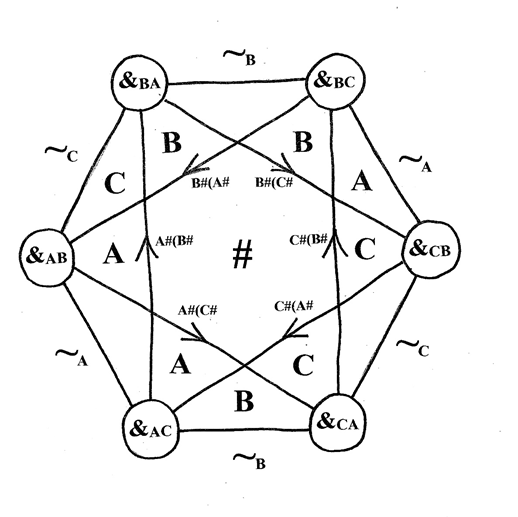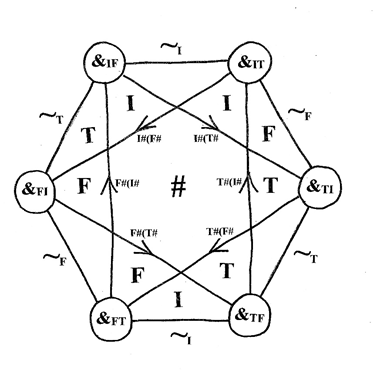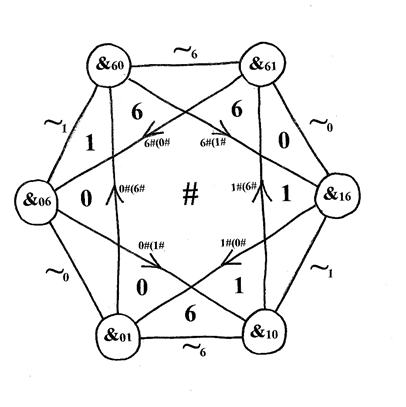## Friday, July 20, 2018

### Laws of Triple Form: 10 of 12

Chapter 9  : Trinary Logics

Majorities
Octohedral distribution
The Hexagram

9A. Majorities

Define three majority operators:
MI(x,y,z)    =   (x &FT y)  &TF (y &FT z)  &TF  (z &FT x)
MT(x,y,z)   =   (x &IF y)  &FI (y &IF z)  &FI  (z &IF x)
MF(x,y,z)   =   (x &IT y)  &TI (y &IT z)  &TI  (z &IT x)

The subscripted majority operator has these identities:

Symmetry:          Ma(x,y,z)  =  Ma(y,z,x)  =  Ma(z,x,y)  =  Ma(x,z,y)
=  Ma(z,y,x)  =  Ma(y,x,z)
Coalition:            Ma(x,x,y)   =   Ma(x,x,x)   =   x
Cancellation:      Ma#b(x, a, b) =       x
Mediocrity:         Mf(f,i,t)  =  f   ;   Mi(f,i,t)  =  i   ;   Mt(f,i,t)  =  t

The subscript ‘a’ is the “Chairman’s Subscript”: it decides three-way ties. These axioms suffice to define all values of Ma(x,y,z);  for either {x,y,z} has three distinct elements (and then Symmetry plus Mediocrity applies) or {x,y,z} has coincidental elements (so Symmetry and Coalition apply).

Permutations:   for any permutation P,   P(Ma(x,y,z))  =  MP(a)(P(x),P(y),P(z))
Subscripts:      x &ab y   =    Ma#b(x, y, a)

Exercise for the student:

Prove that &fi, &ft, &tf, &ti all distribute over Mi; but not &if or &it.
Prove that if a, b and c are three different forms, then
&ac, &ab, &ba, &bc all distribute over Mc; but not &ca or &cb.

9B. Octohedral Distribution

Say that “&ab distributes over &de” if, for every x, y and z from among the three forms:
x  &ab  ( y  &de  z  )    =   (x  &ab  y)  &de  (  x &ab  z  )

There are three cases: {x,y,z} has 1 element, or 2, or 3.

Case 1: {x,y,z} has only 1 element.
Then the equation follows by Recall for each operator:  x&pqx  = x .

Case 2: {x,y,z} has only 2 elements - say, {F,I}.
Then the operators &ab  and  &de  would be min or max operators on the 2-element lattice F<I; these distribute over each other and themselves, therefore &ab  would  distribute over &de.

Case 3: {x,y,z}  =  {F,I,T}.
There are three distinct values for x, y and z. Call them a, b, and c; so there are three cases for x; it equals a, or b, or c.
If X = a, then:
x &ab (y &de z)    =            a &ab (y &de z)    =    a
and
(x &ab y) &de (x &ab z)  =  (a &ab y)&de(a &ab z) = a&dea  = a

If X = b, then:
x &ab (y &de z)    =            b &ab (y &de z)    =    (y &de z)
and
(x &ab y) &de (x &ab z)  =  (b &ab y) &de (b &ab z)  =  (y &de z)

If X = c, then:
x &ab (y &de z)    =            c &ab (y &de z)    =    c &ab (a &de b)
and
( x &ab y)  &de  ( x &ab z )  =  (c &ab a) &de (c &ab b)  =    a &de c
-         because y and z are the other two values a and b.
So now the question is: for what d and e is the following true?
c &ab (a &de b)   =    a &de  c

Just check all six possibilities for d and e:

d        e        c&ab(a&deb)                   a &de c                  check?
-------------------------------------------------------------------------------
a        b        c&ab(a&abb)   = a          a &ab c  = a           yes
a        c        c&ab(a&acb)   = a           a &ac c   = a          yes
b        a        c&ab(a&bab)   = c           a &ba c  = c           yes
b        c        c&ab(a&bcb)   = c           a &bc c  = a           no!
c        a        c&ab(a&cab)   = c            a &ca c  = c           yes
c        b        c&ab(a&cbb)   = a           a &cb c  = c           no!

Note the two exceptions:
c &ab (a&bcb)  = c ;        a &bc c = a
c &ab (a&cbb)  = a ;        a &cb c = c
They are d=b and e=c, or d=c and e=b; in neither case is either d or e equal to a, whereas one of them equals a in every other case.
Therefore this Theorem:
Octohedral Distribution

&ab distributes over &cd
if and only if
a=c   or a=d

9C. The Hexagram

Octohedral distribution is illustrated by the following “distribution octohedron”, a.k.a. the “Hexagram”. Lines join mutually distributing operators, lines with arrows join one-way distributing operators. The pivot a#x is here denoted ~ax; the double-crosses d#(e#x) are denoted d#(e#.The triples (&AC, &BA, &CB) and (&CA, &BC, &AB) display cyclic distribution:

The first distributes over the second;
The second distributes over the third;
The third distributes over the first;
But not the other way around!
Here is the Hexagram in terms of Kleenean logic:Here it is in terms of triple forms: